# 1 NAFEMS LE10 “Thick plate pressure” benchmark

Assuming the CAD has already been created in STEP format (for instance using Gmsh with this geo file), create a tetrahedral locally-refined unstructured grid with Gmsh using the following .geo file:

// NAFEMS LE10 benchmark unstructured locally-refined tetrahedral mesh

// define physical names from the geometrical entity ids
Physical Surface("upper") = {7};
Physical Surface("DCD'C'") = {1};
Physical Surface("ABA'B'") = {3};
Physical Surface("BCB'C'") = {4, 5};
Physical Curve("midplane") = {14};
Physical Volume("bulk") = {1};

// meshing settings, read Gmsh' manual for further reference
Mesh.ElementOrder = 2;      // use second-order tetrahedra
Mesh.Algorithm = 6;         // 2D mesh algorithm:  6: Frontal Delaunay
Mesh.Algorithm3D = 10;      // 3D mesh algorithm: 10: HXT
Mesh.Optimize = 1;          // Optimize the mesh
Mesh.HighOrderOptimize = 1; // Optimize high-order meshes? 2: elastic+optimization

Mesh.MeshSizeMax = 80;      // main element size
Mesh.MeshSizeMin = 20;      // refined element size

// local refinement around the point D (entity 4)
Field = Distance;
Field.NodesList = {4};
Field = Threshold;
Field.IField = 1;
Field.LcMin = Mesh.MeshSizeMin;
Field.LcMax = Mesh.MeshSizeMax;
Field.DistMin = 2 * Mesh.MeshSizeMax;
Field.DistMax = 6 * Mesh.MeshSizeMax;
Background Field = {2};

and then use this pretty-straightforward input file that has a one-to-one correspondence with the original problem formulation from 1990:

# NAFEMS Benchmark LE-10: thick plate pressure
PROBLEM mechanical 3D
READ_MESH nafems-le10.msh   # mesh in millimeters

BC upper    p=1      # 1 Mpa

# BOUNDARY CONDITIONS:
BC DCD'C'   v=0      # Face DCD'C' zero y-displacement
BC ABA'B'   u=0      # Face ABA'B' zero x-displacement
BC BCB'C'   u=0 v=0  # Face BCB'C' x and y displ. fixed
BC midplane w=0      #  z displacements fixed along mid-plane

# MATERIAL PROPERTIES: isotropic single-material properties
E = 210e3   # Young modulus in MPa
nu = 0.3    # Poisson's ratio

SOLVE_PROBLEM   # solve!

# print the direct stress y at D (and nothing more)
PRINT "sigma_y @ D = " sigmay(2000,0,300) "MPa"

# write post-processing data for paraview
WRITE_MESH nafems-le10.vtk sigmay VECTOR u v w
$gmsh -3 nafems-le10.geo [...]$ feenox nafems-le10.fee
sigma_y @ D =   -5.38016        MPa
$Normal stress \sigma_y refined around point D over 5,000x-warped displacements for LE10 created with Paraview # 2 NAFEMS LE11 “Solid Cylinder/Taper/Sphere-Temperature” benchmark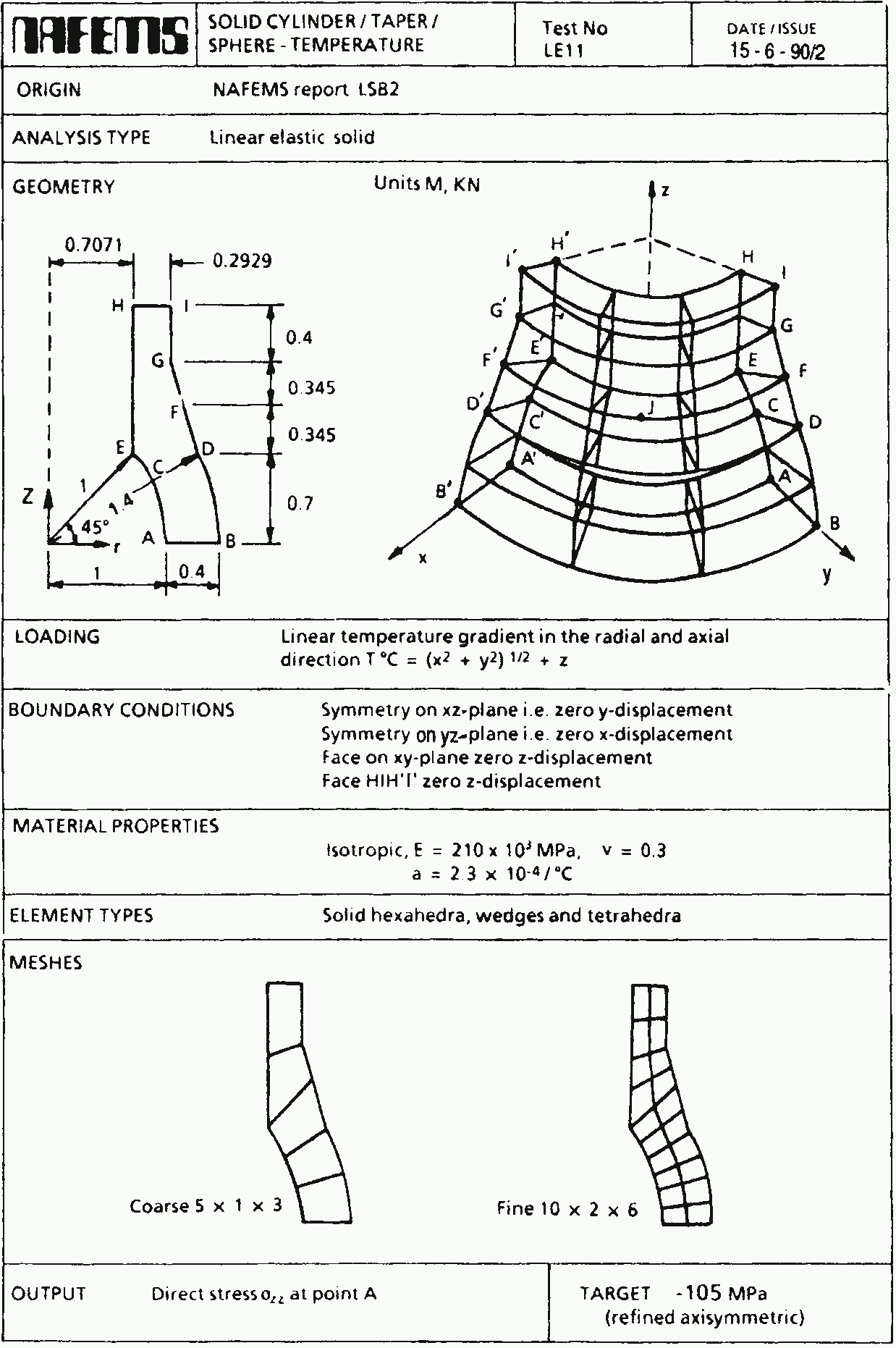Figure 1: The NAFEMS LE11 problem formulation. a — Problem statement, b — Structured hex mesh Following the spirit from LE10, note how easy it is to give a space-dependent temperature field in FeenoX. Just write \sqrt{x^2+y^2}+z like sqrt(x^2 + y^2) + z! # NAFEMS Benchmark LE-11: solid cylinder/taper/sphere-temperature PROBLEM mechanical 3D READ_MESH nafems-le11.msh # linear temperature gradient in the radial and axial direction # as an algebraic expression as human-friendly as it can be T(x,y,z) := sqrt(x^2 + y^2) + z BC xz v=0 # displacement vector is [u,v,w] BC yz u=0 # u = displacement in x BC xy w=0 # v = displacement in y BC HIH'I' w=0 # w = displacement in z E = 210e3*1e6 # mesh is in meters, so E=210e3 MPa -> Pa nu = 0.3 # dimensionless alpha = 2.3e-4 # in 1/ºC as in the problem SOLVE_PROBLEM # for post-processing in Paraview WRITE_MESH nafems-le11.vtk VECTOR u v w T sigmax sigmay sigmaz PRINT "sigma_z(A) =" %.2f sigmaz(1,0,0)/1e6 "MPa" SEP " " PRINT "wall time =" %.2f wall_time() "seconds" SEP " " $ gmsh -3 nafems-le11.geo
[...]
$feenox nafems-le11.fee sigma_z(A) = -105.04 MPa wall time = wall time = 1.91 seconds$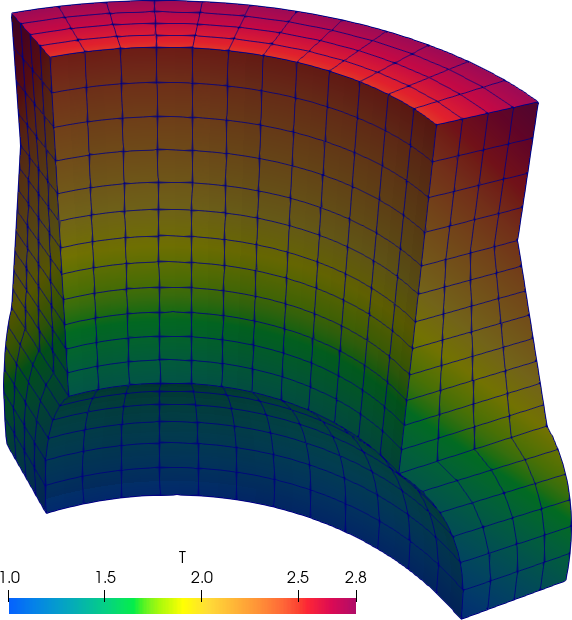Figure 2: The NAFEMS LE11 problem results. a — Problem statement, b — Structured hex mesh

# 3 NAFEMS LE1 “Elliptical membrane” plane-stress benchmark

Tell FenooX the problem is plane_stress. Use the nafems-le1.geo file provided to create the mesh. Read it with READ_MESH, set material properties, BCs and SOLVE_PROBLEM!

PROBLEM mechanical plane_stress

E = 210e3
nu = 0.3

BC AB u=0
BC CD v=0
BC BC tension=10

SOLVE_PROBLEM

WRITE_MESH nafems-le1.vtk VECTOR u v 0 sigmax sigmay tauxy
PRINT "σy at point D = " %.4f sigmay(2000,0) "(reference is 92.7)" SEP " "
$gmsh -2 nafems-le11.geo [...]$ feenox nafems-le1.fee
σy at point D =  92.7011 (reference is 92.7)
$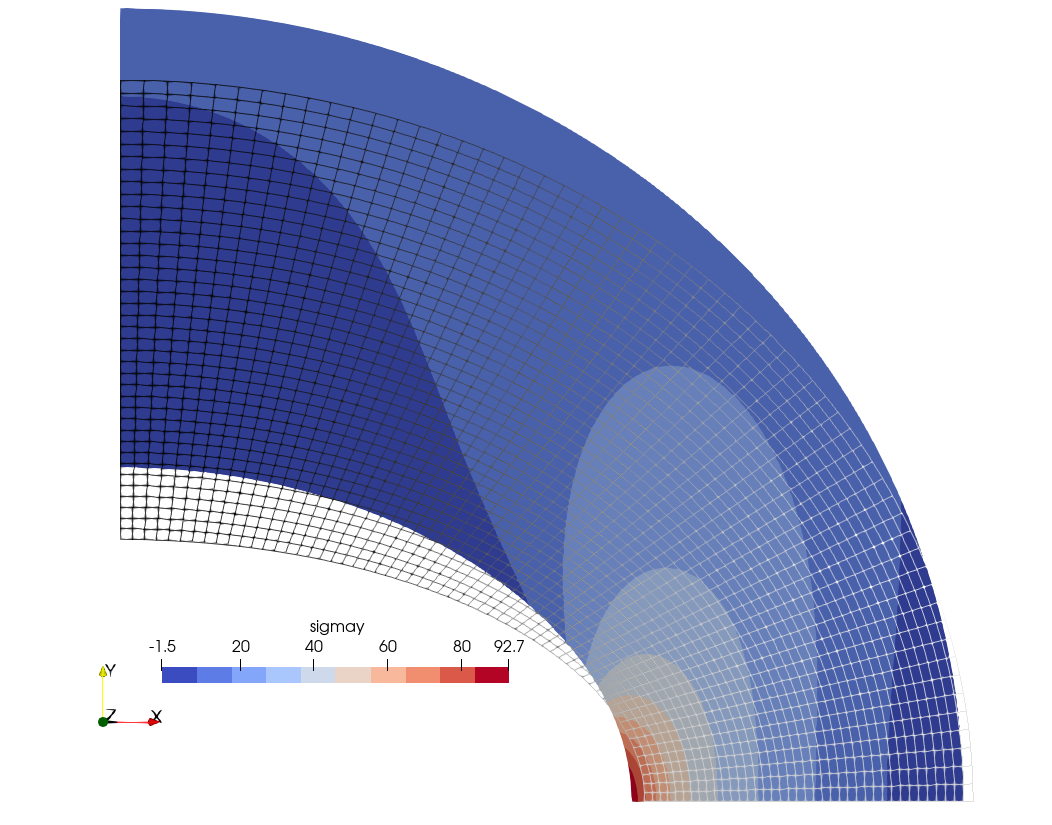Normal stress \sigma_y over 500x-warped displacements for LE1 created with Paraview # 4 Parametric study on a cantilevered beam If an external loop successively calls FeenoX with extra command-line arguments, a parametric run is obtained. This file cantilever.fee fixes the face called “left” and sets a load in the negative z direction of a mesh called cantilever-$1-$2.msh, where $1 is the first argument after the inpt file and $2 the second one. The output is a single line containing the number of nodes of the mesh and the displacement in the vertical direction w(500,0,0) at the center of the cantilever’s free face. The following Bash script first calls Gmsh to create the meshes. To do so, it first starts with a base cantilever.geo file that creates the CAD: // https://autofem.com/examples/determining_natural_frequencie.html SetFactory("OpenCASCADE"); L = 0.5; b = 0.05; h = 0.02; Box(1) = {0,-b/2,-h/2, L, b, h}; Physical Surface("left") = {1}; Physical Surface("right") = {2}; Physical Surface("top") = {4}; Physical Volume("bulk") = {1}; Transfinite Curve {1, 3, 5, 7} = 1/(Mesh.MeshSizeFactor*Mesh.ElementOrder) + 1; Transfinite Curve {2, 4, 6, 8} = 2/(Mesh.MeshSizeFactor*Mesh.ElementOrder) + 1; Transfinite Curve {9, 10, 11, 12} = 16/(Mesh.MeshSizeFactor*Mesh.ElementOrder) + 1; Transfinite Surface "*"; Transfinite Volume "*"; Then another .geo file is merged to build cantilever-${element}-${c}.msh where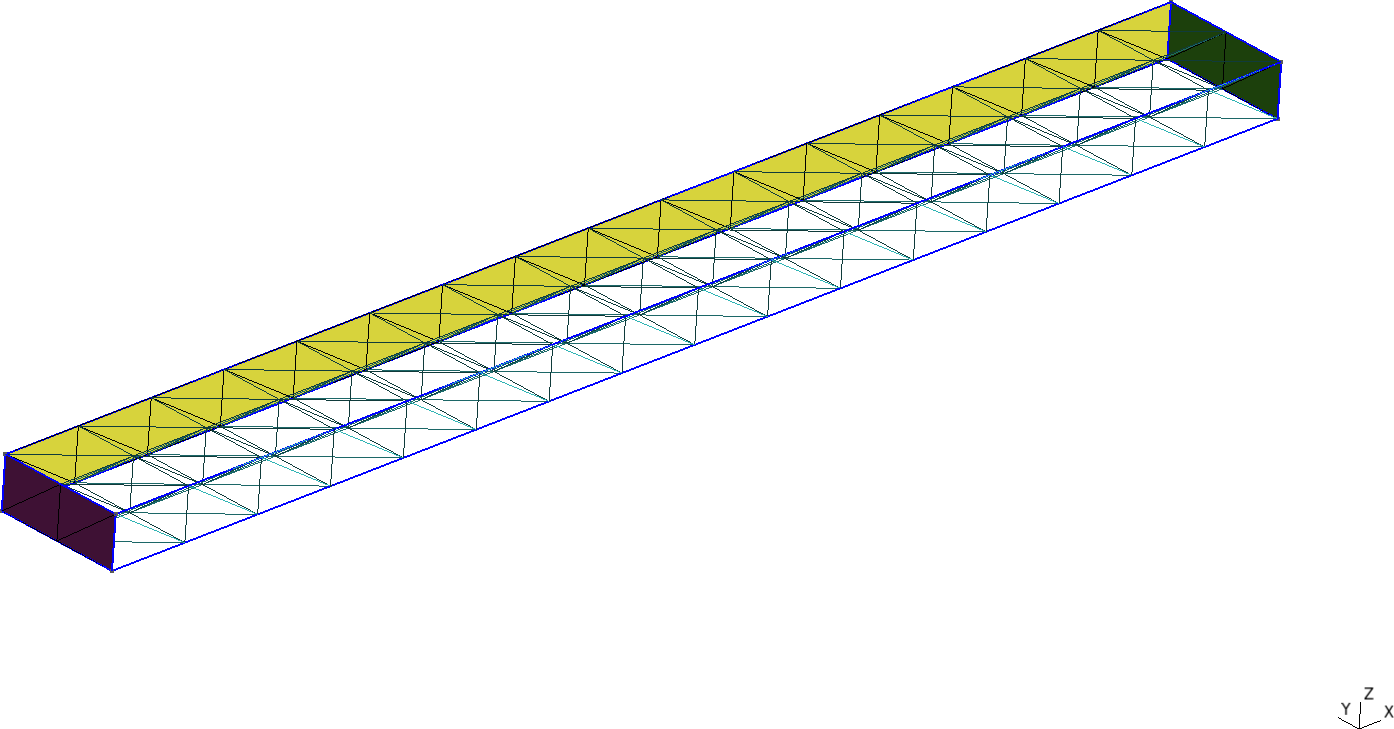Figure 3: Cantilevered beam meshed with structured tetrahedra and hexahedra. a — Tetrahedra, b — Hexahedra It then calls FeenoX with the input cantilever.fee and passes ${element} and ${c} as extra arguments, which then are expanded as $1 and $2 respectively. #!/bin/bash rm -f *.dat for element in tet4 tet10 hex8 hex20 hex27; do for c in$(seq 1 10); do

# create mesh if not alreay cached
mesh=cantilever-${element}-${c}
if [ ! -e ${mesh}.msh ]; then scale=$(echo "PRINT 1/${c}" | feenox -) gmsh -3 -v 0 cantilever-${element}.geo -clscale ${scale} -o${mesh}.msh
fi

# call FeenoX
feenox cantilever.fee ${element}${c} | tee -a cantilever-${element}.dat done done After the execution of the Bash script, thanks to the design decision that output is 100% defined by the user (in this case with the PRINT instruction), one has several files cantilever-${element}.dat files. When plotted, these show the shear locking effect of fully-integrated first-order elements. The theoretical Euler-Bernoulli result is just a reference as, among other things, it does not take into account the effect of the material’s Poisson’s ratio. Note that the abscissa shows the number of nodes, which are proportional to the number of degrees of freedom (i.e. the size of the problem matrix) and not the number of elements, which is irrelevant here and in most problems.

PROBLEM mechanical 3D
READ_MESH cantilever-$1-$2.msh   # in meters

E = 2.1e11         # Young modulus in Pascals
nu = 0.3           # Poisson's ratio

BC left   fixed
BC right  tz=-1e5  # traction in Pascals, negative z

SOLVE_PROBLEM

# z-displacement (components are u,v,w) at the tip vs. number of nodes
PRINT nodes %e w(500,0,0) "\# $1$2"
$./cantilever.sh 102 -7.641572e-05 # tet4 1 495 -2.047389e-04 # tet4 2 1372 -3.149658e-04 # tet4 3 [...] 19737 -5.916234e-04 # hex27 8 24795 -5.916724e-04 # hex27 9 37191 -5.917163e-04 # hex27 10$ pyxplot cantilever.pplDisplacement at the free tip of a cantilevered beam vs. number of nodes for different element types

# 5 Parallelepiped whose Young’s modulus is a function of the temperature

The problem consists of finding the non-dimensional temperature T and displacements u, v and w distributions within a solid parallelepiped of length l whose base is a square of h\times h. The solid is subject to heat fluxes and to a traction pressure at the same time. The non-dimensional Young’s modulus E of the material depends on the temperature T in a know algebraically way, whilst both the Poisson coefficient \nu and the thermal conductivity k are uniform and do not depend on the spatial coordinates:

\begin{aligned} E(T) &= \frac{1000}{800-T} \\ \nu &= 0.3 \\ k &= 1 \\ \end{aligned}

References:

This thermo-mechanical problem is solved in two stages. First, the heat conduction equation is solved over a coarse first-order mesh to find the non-dimensional temperature distribution. Then, a mechanical problem is solved where T(x,y,z) is read from the first mesh and interpolated into a finer second-order mesh so to as evaluate the non-dimensional Young’s modulus as

E\Big(T(x,y,z)\Big) = \frac{1000}{800-T(x,y,z)}

Note that there are not thermal expansion effects (i.e. the thermal expansion coefficient is \alpha=0). Yet, suprinsingly enough, the problem has analytical solutions for both the temperature and the displacement fields.

## 5.1 Thermal problem

The following input solves the thermal problem over a coarse first-order mesh, writes the resulting temperature distribution into parallelepiped-temperature.msh, and prints the L_2 error of the numerical result with respect to the analytical solution T(x,y,z) = 40 - 2x - 3y - 4z.

PROBLEM thermal 3D

k = 1     # unitary non-dimensional thermal conductivity

# boundary conditions
BC left    q=+2
BC right   q=-2
BC front   q=+3
BC back    q=-3
BC bottom  q=+4
BC top     q=-4
BC A       T=0

SOLVE_PROBLEM
WRITE_MESH parallelepiped-temperature.msh T

# compute the L-2 norm of the error in the displacement field
Te(x,y,z) = 40 - 2*x - 3*y - 4*z   # analytical solution, "e" means exact
INTEGRATE (T(x,y,z)-Te(x,y,z))^2 RESULT num
PHYSICAL_GROUP bulk DIM 3  # this is just to compute the volume
PRINT num/bulk_volume
$gmsh -3 parallelepiped.geo -order 1 -clscale 2 -o parallelepiped-coarse.msh [...] Info : 117 nodes 567 elements Info : Writing 'parallelepiped-coarse.msh'... Info : Done writing 'parallelepiped-coarse.msh' Info : Stopped on Fri Dec 10 10:32:30 2021 (From start: Wall 0.0386516s, CPU 0.183052s)$ feenox parallelepiped-thermal.fee
6.18981e-12
$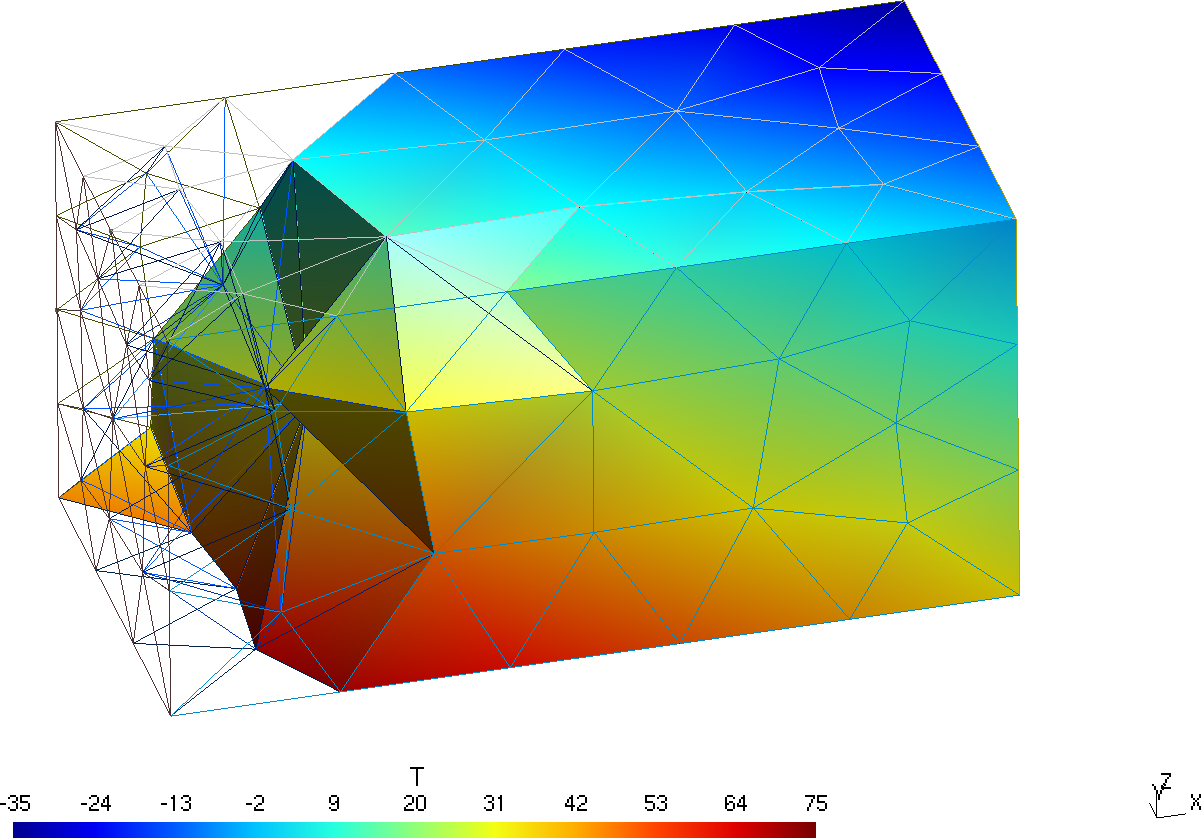Temperature distribution over the coarse mesh in Gmsh (yes, it is a rainbow pallete) ## 5.2 Mechanical problem Now this input file reads the scalar function T stored in the coarse first-order mesh file parallelepiped-temperature.msh and uses it to solve the mechanical problem in the finer second-order mesh parallelepiped.msh. The numerical solution for the displacements over the fine mesh is written in a VTK file (along with the temperature as interpolated from the coarse mesh) and compared to the analytical solution using the L_2 norm. PROBLEM mechanical 3D # this is where we solve the mechanical problem READ_MESH parallelepiped.msh MAIN # this is where we read the temperature from READ_MESH parallelepiped-temperature.msh DIM 3 READ_FUNCTION T # mechanical properties E(x,y,z) = 1000/(800-T(x,y,z)) # young's modulus nu = 0.3 # poisson's ratio # boundary conditions BC O fixed BC B u=0 w=0 BC C u=0 # here "load" is a fantasy name applied to both "left" and "right" BC load tension=1 PHYSICAL_GROUP left PHYSICAL_GROUP right SOLVE_PROBLEM WRITE_MESH parallelepiped-mechanical.vtk T VECTOR u v w # analytical solutions h = 10 A = 0.002 B = 0.003 C = 0.004 D = 0.76 # the "e" means exact ue(x,y,z) := A/2*(x^2 + nu*(y^2+z^2)) + B*x*y + C*x*z + D*x - nu*A*h/4*(y+z) ve(x,y,z) := -nu*(A*x*y + B/2*(y^2-z^2+x^2/nu) + C*y*z + D*y -A*h/4*x - C*h/4*z) we(x,y,z) := -nu*(A*x*z + B*y*z + C/2*(z^2-y^2+x^2/nu) + D*z + C*h/4*y - A*h/4*x) # compute the L-2 norm of the error in the displacement field INTEGRATE (u(x,y,z)-ue(x,y,z))^2+(v(x,y,z)-ve(x,y,z))^2+(w(x,y,z)-we(x,y,z))^2 RESULT num MESH parallelepiped.msh INTEGRATE 1 RESULT den MESH parallelepiped.msh PRINT num/den $ gmsh -3 parallelepiped.geo -order 2
[...]
Info    : 2564 nodes 2162 elements
Info    : Writing 'parallelepiped.msh'...
Info    : Done writing 'parallelepiped.msh'
Info    : Stopped on Fri Dec 10 10:39:27 2021 (From start: Wall 0.165707s, CPU 0.258751s)
$feenox parallelepiped-mechanical.fee 1.46196e-06$Displacements and temperature distribution over the fine mesh in Paraview (yes, still a rainbow pallete)

# 6 Orthotropic free expansion of a cube

To illustrate the point of the previous discussion, let us solve the thermal expansion of an unrestrained unitary cube [0,1~\text{mm}]\times[0,1~\text{mm}]\times[0,1~\text{mm}] subject to a linear radially-symmetric temperature field T(x,y,z) = 30 \text{ºC} + 150 \frac{\text{ºC}}{\text{mm}} \sqrt{x^2+y^2+z^2}

with a mean thermal expansion coefficient for each of the three directions x, y and z computed from each of the three columns of the ASME table TE-2, respectively. If the data was consistent, the displacement at any point with the same coordinates x=y=z would be exactly equal.

DEFAULT_ARGUMENT_VALUE 1 steffen
DEFAULT_ARGUMENT_VALUE 2 hex

PROBLEM mechanical
READ_MESH cube-$2.msh # aluminum-like linear isotropic material properties E = 69e3 nu = 0.28 # free expansion BC left u=0 BC front v=0 BC bottom w=0 # reference temperature is 20ºC T0 = 20 # spatial temperature distribution symmetric wrt x,y & z T(x,y,z) = 30+150*sqrt(x^2+y^2+z^2) # read ASME data FUNCTION A(T') FILE asme-expansion-table.dat COLUMNS 1 2 INTERPOLATION$1
FUNCTION B(T') FILE asme-expansion-table.dat COLUMNS 1 3 INTERPOLATION $1 FUNCTION C(T') FILE asme-expansion-table.dat COLUMNS 1 4 INTERPOLATION$1

# remember that the thermal expansion coefficients have to be
#  1. the mean value between T0 and T
#  2. functions of space, so temperature has to be written as T(x,y,z)

# in the x direction, we use column B directly
alpha_x(x,y,z) = 1e-6*B(T(x,y,z))

# in the y direction, we convert column A to mean
alpha_y(x,y,z) = 1e-6*integral(A(T'), T', T0, T(x,y,z))/(T(x,y,z)-T0)

# in the z direction, we convert column C to mean
alpha_z(x,y,z) = 1e-3*C(T(x,y,z))/(T(x,y,z)-T0)

SOLVE_PROBLEM

WRITE_MESH cube-orthotropic-expansion-$1-$2.vtk T VECTOR u v w
PRINT %.3e "displacement in x at (1,1,1) = " u(1,1,1)
PRINT %.3e "displacement in y at (1,1,1) = " v(1,1,1)
PRINT %.3e "displacement in z at (1,1,1) = " w(1,1,1)
$gmsh -3 cube-hex.geo [...]$ gmsh -3 cube-tet.geo
[...]
$feenox cube-orthotropic-expansion.fee displacement in x at (1,1,1) = 4.451e-03 displacement in y at (1,1,1) = 4.449e-03 displacement in z at (1,1,1) = 4.437e-03$ feenox cube-orthotropic-expansion.fee linear tet
displacement in x at (1,1,1) =  4.452e-03
displacement in y at (1,1,1) =  4.447e-03
displacement in z at (1,1,1) =  4.438e-03
$feenox cube-orthotropic-expansion.fee akima hex displacement in x at (1,1,1) = 4.451e-03 displacement in y at (1,1,1) = 4.451e-03 displacement in z at (1,1,1) = 4.437e-03$ feenox cube-orthotropic-expansion.fee splines tet
displacement in x at (1,1,1) =  4.451e-03
displacement in y at (1,1,1) =  4.450e-03
displacement in z at (1,1,1) =  4.438e-03
$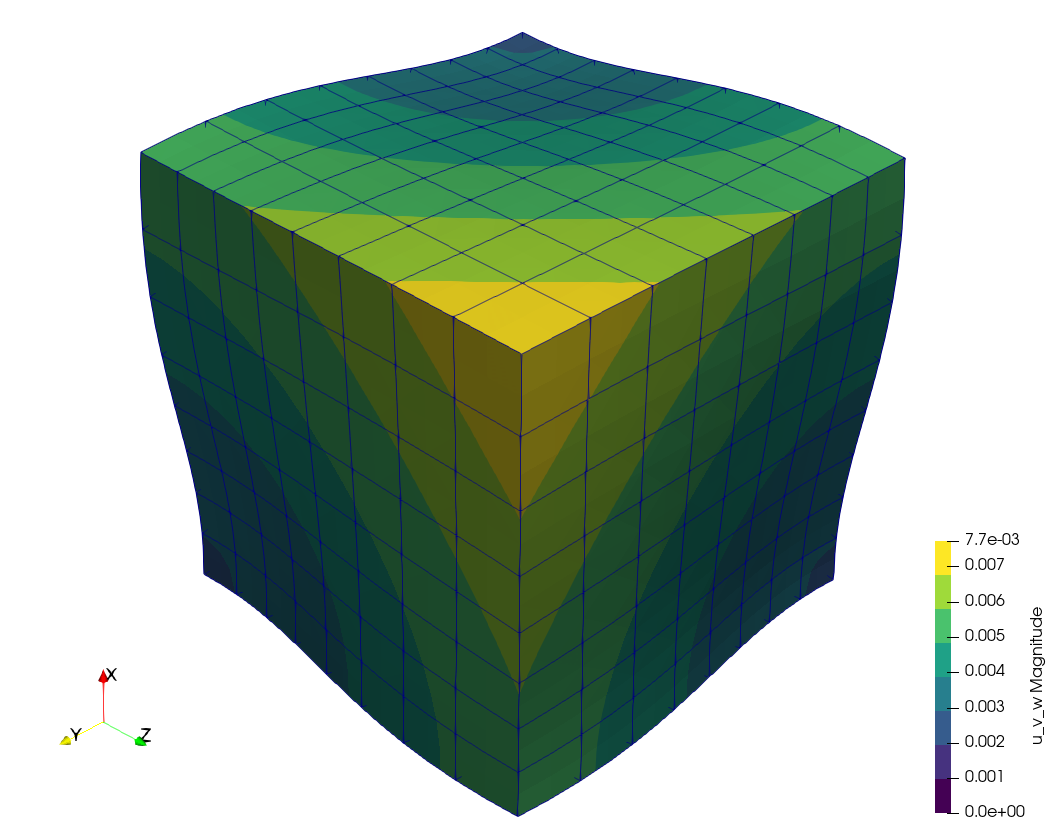Warped displacement (\times 500) of the cube using ASME’s three columns. Differences cannot be seen graphically, but they are there as the terminal mimic illustrates. Yet, they are not as large nor as sensible to meshing and interpolation settings as one would have expected after seeing the plots from the previous section. # 7 Thermo-elastic expansion of finite cylinders Let us solve the following problem introduced by J. Veeder in his technical report AECL-2660 from 1967.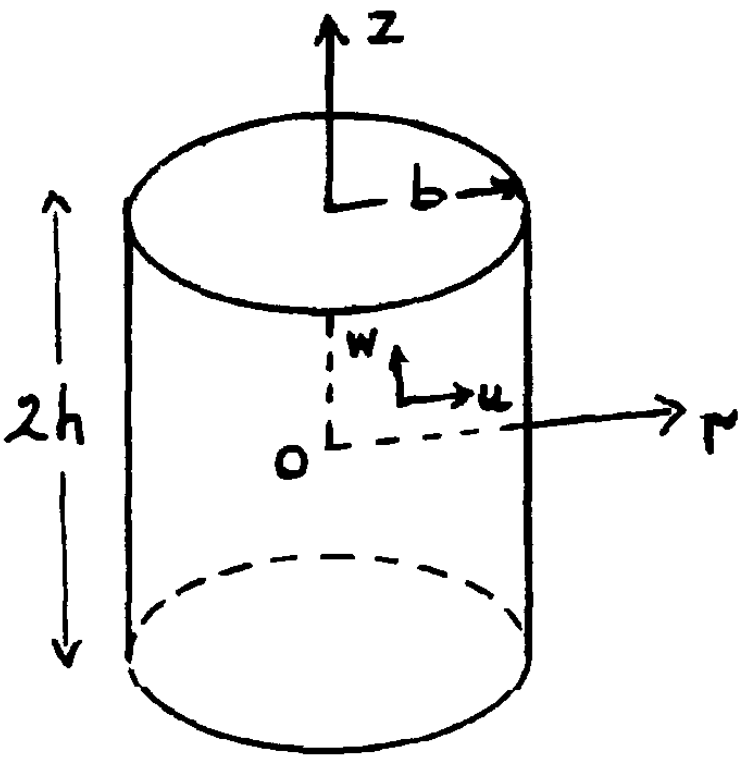Consider a finite solid cylinder (see insert) of radius b and length 2h, with the origin of coordinates at the centre. It may be shown that the temperature distribution in a cylindrical fuel pellet operating in a reactor is given approximately by T(r) = T_0 + T_1 \cdot \left[ 1 - \left(\frac{r}{b} \right)^2 \right] where T_0 is the pellet surface temperature and T_1 is the temperature difference between the centre and surface. The thermal expansion is thus seen to be the sum of two terms, the first of which produces uniform expansion (zero stress) at constant temperature T_0, and is therefore computationally trivial. Tho second term introduces non-uniform body forces which distort the pellet from its original cylindrical shape. The problem is axisymmetric on the azimutal angle and axially-symmetric along the mid-plane. The FeenoX input uses the tangential and radial boundary conditions applied to the base of the upper half of a 3D cylinder. The geometry is meshed using 27-noded hexahedra. Two one-dimensional profiles for the non-dimensional range [0:1] at the external surfaces are written into an ASCII file ready to be plotted: 1. axial dependency of the displacement v(z') = v(0,v,z'h) in the y direction at fixed x=0 and y=b, and 2. radial dependency of the displacement w(r') = w(0,r'b, h) in the z direction at fixed x=0 and z=h These two profiles are compared to the power expansion series given in the original report from 1967. Note that the authors expect a 5% difference between the reference solution and the real one.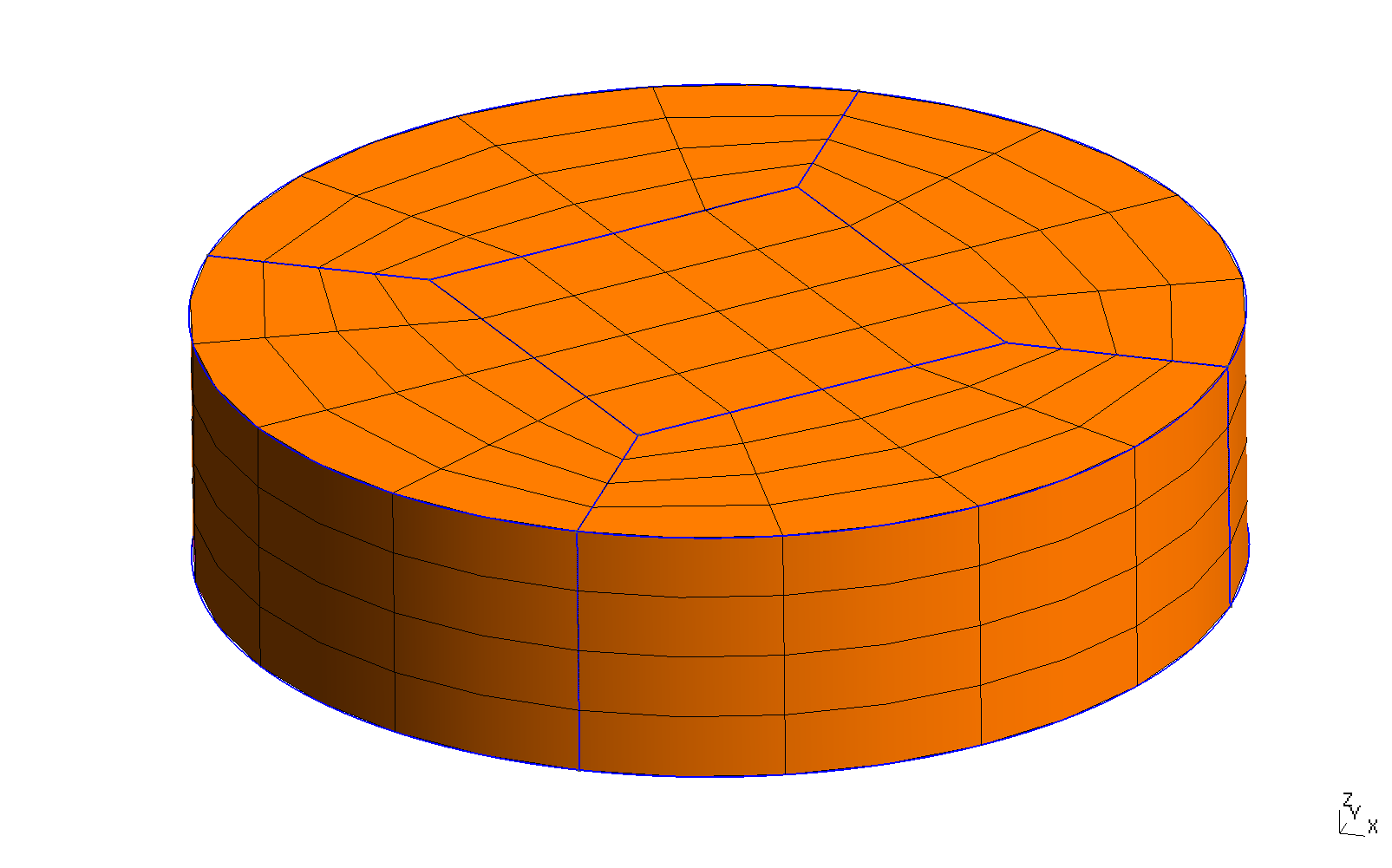3D mesh of the upper half of the Veeder problem PROBLEM mechanical READ_MESH veeder.msh b = 1 # cylinder radius h = 0.5 # cylinder height E = 1 # young modulus (does not matter for the displacement, only for stresses) nu = 1/3 # poisson ratio alpha = 1e-5 # temperature expansion coefficient # temperature distribution as in the original paper T1 = 1 # maximum temperature T0 = 0 # reference temperature (where expansion is zero) T(x,y,z) := T0 + T1*(1-(x^2+y^2)/(b^2)) # boundary conditions (note that the cylinder can still expand on the x-y plane) BC inf tangential radial # solve! SOLVE_PROBLEM # write vtk output WRITE_MESH veeder.vtk T sigma dudx dudy dudz dvdx dvdy dvdz dwdx dwdy dwdz sigma1 sigma2 sigma3 VECTOR u v w # non-dimensional numerical displacement profiles v_num(z') = v(0, b, z'*h)/(alpha*T1*b) w_num(r') = w(0, r'*b, h)/(alpha*T1*b) ######## # reference solution # coefficients of displacement functions for h/b = 0.5 a00 = 0.66056 a01 = -0.44037 a10 = 0.23356 a02 = -0.06945 a11 = -0.10417 a20 = 0.00288 b00 = -0.01773 b01 = -0.46713 b10 = -0.04618 b02 = +0.10417 b11 = -0.01152 b20 = -0.00086 # coefficients of displacement functions for h/b = 1.0 # a00 = 0.73197 # a01 = -0.48798 # a10 = 0.45680 # a02 = -0.01140 # a11 = -0.06841 # a20 = 0.13611 # # b00 = 0.26941 # b01 = -0.45680 # b10 = -0.25670 # b02 = 0.03420 # b11 = -0.27222 # b20 = -0.08167 R(r') = r'^2 - 1 Z(z') = z'^2 - 1 v_ref(r',z') = r' * (a00 + a01*R(r') + a10*Z(z') + a02* R(r')^2 + a11 * R(r')*Z(z') + a20 * Z(z')^2) w_ref(r',z') = z' * (b00 + b01*R(r') + b10*Z(z') + b02* R(r')^2 + b11 * R(r')*Z(z') + b20 * Z(z')^2) PRINT_FUNCTION FILE veeder_v.dat v_num v_ref(1,z') MIN 0 MAX 1 NSTEPS 50 HEADER PRINT_FUNCTION FILE veeder_w.dat w_num w_ref(r',1) MIN 0 MAX 1 NSTEPS 50 HEADER $ gmsh -3 veeder.geo
[...]
$feenox veeder.fee$ pyxplot veeder.ppl
$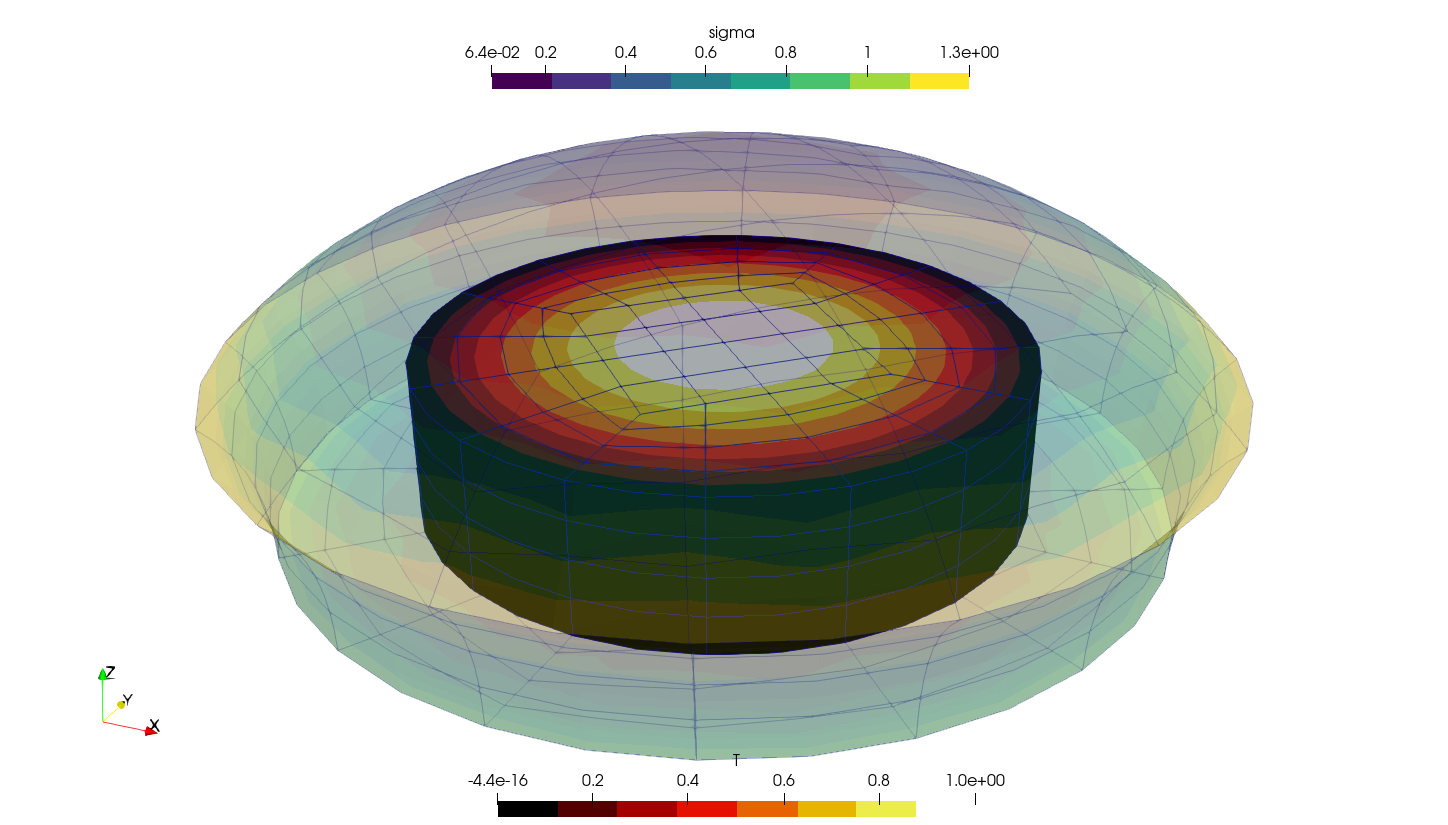100,000x-warped displacementsComparison of 1-D displacement profiles # 8 Temperature-dependent material properties Let us solve a plane-strain square fixed on the left, with an horizontal traction on the right and free on the other two sides. The Young modulus depends on the temperature E(T) as given in the ASME II part D tables of material properties, interpolated using a monotonic cubic scheme. Actually, this example shows three cases: 1. Uniform temperature indentically equal to 200ºC 2. Linear temperature profile on the vertical direction given by an algebraic expression T(x,y) = 200 + 350\cdot y 3. The same linear profile but read from the output of a thermal conduction problem over a non-conformal mesh using this FeenoX input: PROBLEM thermal 2D READ_MESH square-centered-unstruct.msh # [-1:+1]x[-1:+1] BC bottom T=-150 BC top T=+550 k = 1 SOLVE_PROBLEM WRITE_MESH thermal-square-temperature.msh T Which of the three cases is executed is given by the first argument provided in the command line after the main input file. Depending on this argument, which is expanded as $1 in the main input file, either one of three secondary input files are included:

1. uniform

# uniform
T(x,y) := 200
2. linear

# algebraic expression
T(x,y) := 200 + 350*y
3. mesh

# read the temperature from a previous result
READ_MESH thermal-square-temperature.msh DIM 2 READ_FUNCTION T
# 2d plane strain mechanical problem over the [-1:+1]x[-1:+1] square
PROBLEM mechanical plane_strain

# fixed at left, uniform traction in the x direction at right
BC left    fixed
BC right   tx=50

# ASME II Part D pag. 785 Carbon steels with C<=0.30%
FUNCTION E_carbon(temp) INTERPOLATION steffen DATA {
-200  216
-125  212
-75   209
25    202
100   198
150   195
200   192
250   189
300   185
350   179
400   171
450   162
500   151
550   137
}

# read the temperature according to the run-time argument $1 INCLUDE mechanical-square-temperature-$1.fee

# Young modulus is the function above evaluated at the local temperature
E(x,y) := E_carbon(T(x,y))

# uniform Poisson's ratio
nu = 0.3

SOLVE_PROBLEM
PRINT u(1,1) v(1,1)
WRITE_MESH mechanical-square-temperature-$1.vtk E T VECTOR u v 0  $ gmsh -2 square-centered.geo
[...]
Info    : Done meshing 2D (Wall 0.00117144s, CPU 0.00373s)
Info    : 1089 nodes 1156 elements
Info    : Writing 'square-centered.msh'...
Info    : Done writing 'square-centered.msh'
Info    : Stopped on Thu Aug  4 09:40:09 2022 (From start: Wall 0.00818854s, CPU 0.031239s)
$feenox mechanical-square-temperature.fee uniform 0.465632 -0.105128$ feenox mechanical-square-temperature.fee linear
0.589859        -0.216061
$gmsh -2 square-centered-unstruct.geo [...] Info : Done meshing 2D (Wall 0.0274833s, CPU 0.061072s) Info : 65 nodes 132 elements Info : Writing 'square-centered-unstruct.msh'... Info : Done writing 'square-centered-unstruct.msh' Info : Stopped on Sun Aug 7 18:33:41 2022 (From start: Wall 0.0401667s, CPU 0.107659s)$ feenox thermal-square.fee
$feenox mechanical-square-temperature.fee mesh 0.589859 -0.216061$


# 9 Two cubes compressing each other

Say we have two cubes of non-dimensional size 1\times 1 \times 1, one made out of a (non-dimensional) “soft” material and one made out of a “hard” material. We glue the two cubes together, set radial and tangential symmetry conditions on one side of the soft cube (so as to allow pure traction conditions) and set a normal compressive pressure at the other end on the hard cube. Besides on single VTK file with the overall results, the von Mises stress output is split into two VTK files—namely soft.vtk and hard.vtk where the stress is non-zero only in the corresponding volume.

This example illustrates how to

1. assign different material properties to different volumes
2. write VTK outputs segmented by mesh volumes
3. write results at nodes (default) and at cells
PROBLEM mechanical 3D

MATERIAL left   E=1   nu=0.35  mat=1
MATERIAL right  E=10  nu=0.25  mat=2

# BCs
BC ramp  p=0.25

SOLVE_PROBLEM

sigma_at_1(x,y,z) = sigma(x,y,z)*(mat(x,y,z)=1)
sigma_at_2(x,y,z) = sigma(x,y,z)*(mat(x,y,z)=2)

WRITE_RESULTS format vtk displacements vonmises
WRITE_MESH two-cubes-left.vtk  sigma_at_1 CELL NAME sigma_at_1_cells sigma_at_1
WRITE_MESH two-cubes-right.vtk sigma_at_2 CELL NAME sigma_at_2_cells sigma_at_2
$gmsh -3 two-cubes.geo -order 2 -o two-cubes2.msh [...]$ feenox two-cubes-mechanical.fee --mumps
\$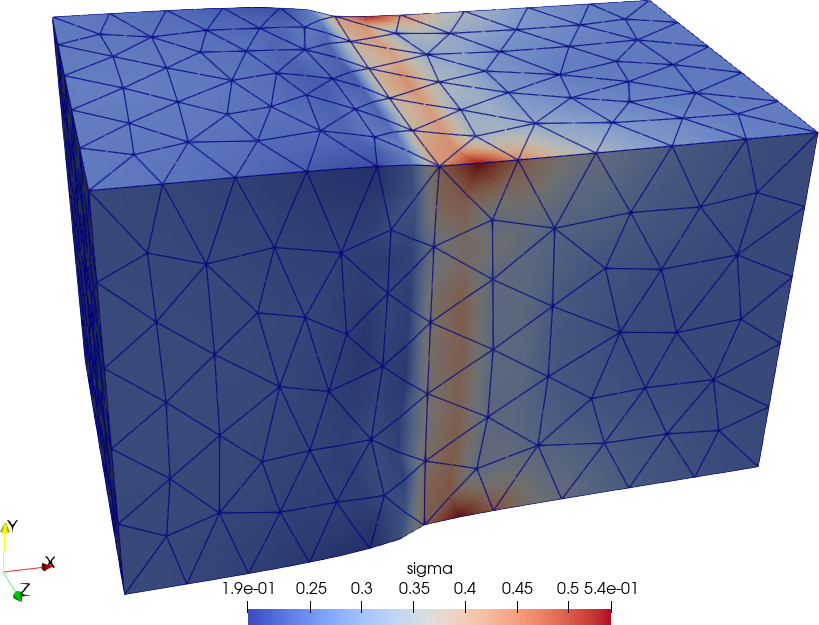Displacements and von Mises stresses from WRITE_RESULTS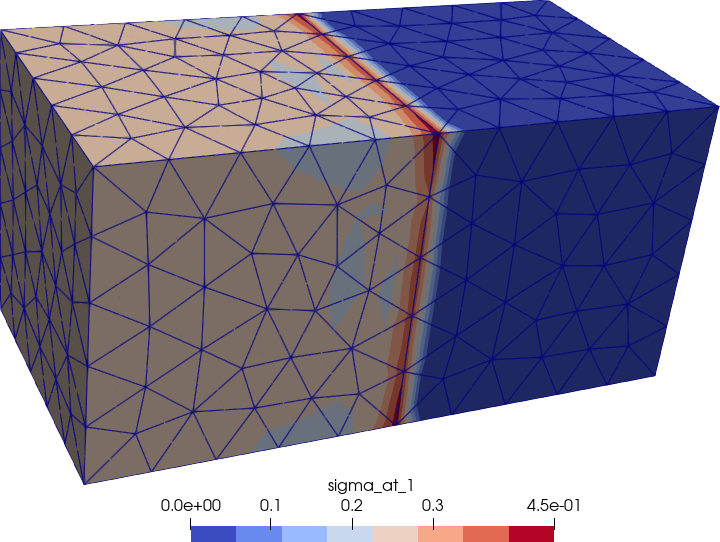Figure 5: Von Mises stresses non-zero only over the left (soft) cube.. a — Data at nodes, b — Data at cells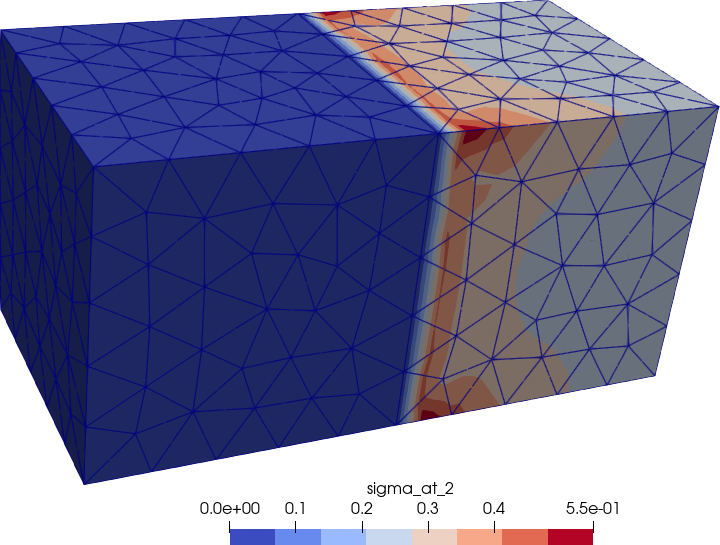Figure 6: Von Mises stresses non-zero only over the right (hard) cube.. a — Data at nodes, b — Data at cells